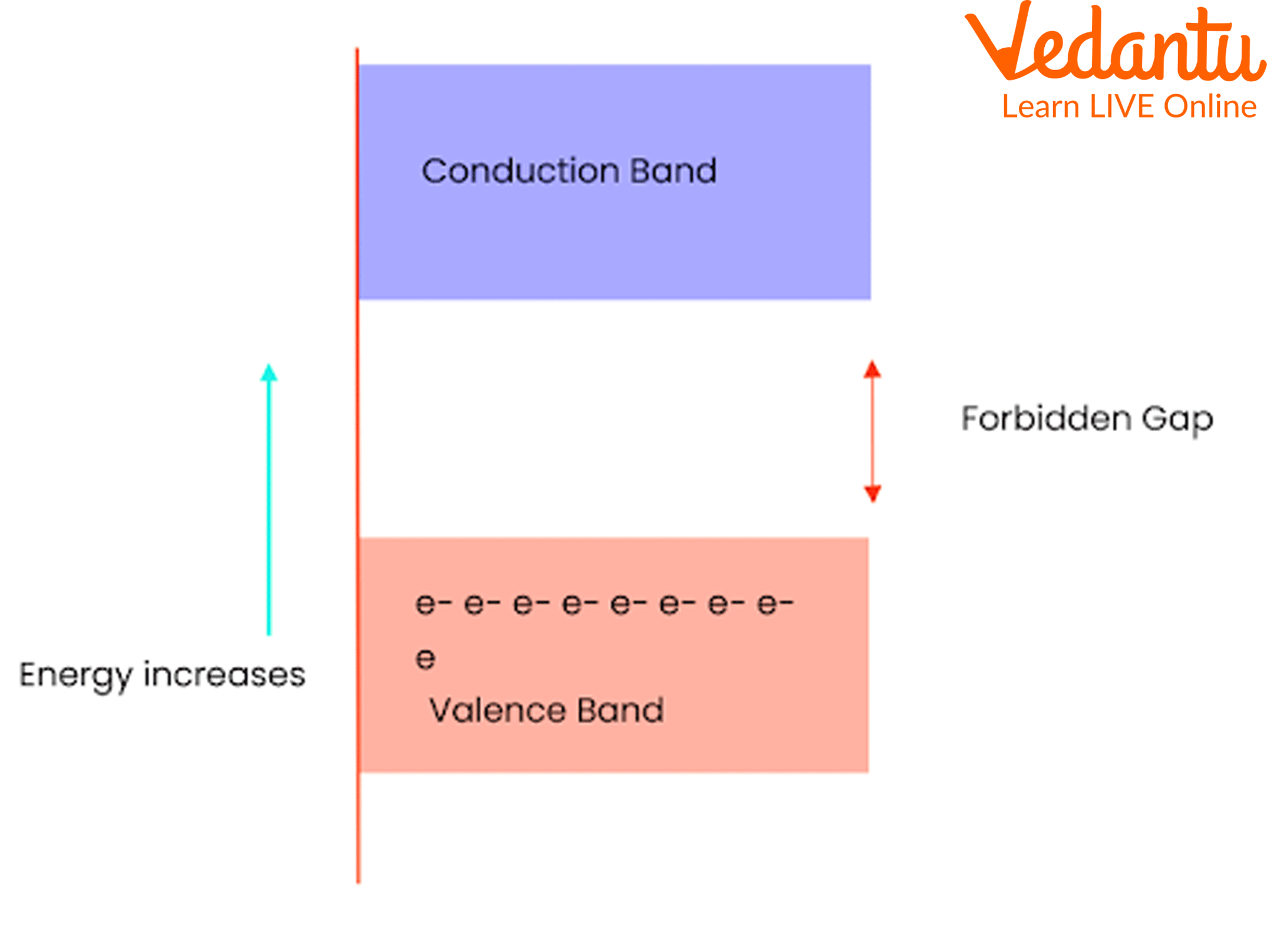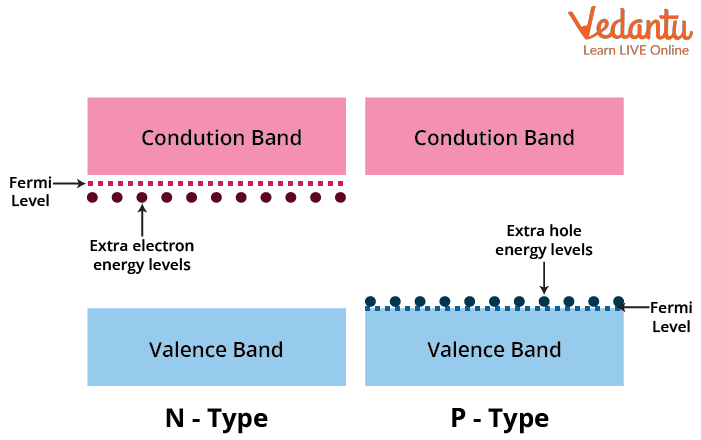Courses
Courses for Kids
Free study material
Free LIVE classes
More

# Energy Bands - Important Concepts for JEELIVE
Join Vedantu’s FREE Mastercalss

## An Introduction to Energy Bands

In Physics, we have already studied substances like solids and fluids, where fluids include both liquids and gases. Also, we know that the movement of particles in gases is the most separated and thus the particles are able to move freely. This movement of molecules is higher in gases as the bonds between them are not stronger. When it comes to liquids, the bonds between the liquid molecules get a little stronger and this results in a not-so-free movement of particles. In contrast, the separation of atoms from their bonds is very hard because there are molecules which occupy a position even in the orbitals of other atoms which lie in the neighbourhood. This is why the orbitals in which electrons are moving overlap with each other due to tight bonding in solids.

Let's take a case where we have only a single atom. It certainly possesses some energy as it has an electron in its orbit. However, when there are two or more atoms bonded together, there will be overlapping between the orbitals where the electrons are present in the outermost shell, which we term as valence shell. This intermixing of electrons in the valence shells of the atoms results in the formation of several energy levels, which are eventually termed as energy bands. Let us know what energy band is clearly in this article.

## What is an Energy Band?

As we already discussed, when there is an overlap between atoms due to tight bonding in solids, the electrons which are present in the outermost shell share positions with the outer shells of other atoms. This is why the energy of electrons present in the valence shell of one atom changes due to the surrounding electron orbits. The reason why the electron is affected by the surrounding atoms is that the outermost electron is the one which is near to the nucleus of its neighbouring atom.

As nuclei have protons which carry a positive charge, this leads to the attraction between the valence electrons and the nucleus. Therefore, the energy levels of the electron keep changing due to this reason. There is an important theory called the energy band theory which was invented by Bohr and is often called the Bohr's theory.

The energy band theory states that there are different energy levels at which every shell of an atom constitutes a distinct amount of energy. This theory is defined to convey the communication between the electrons in the inside shell and the outer shells. Also, even this theory is the foundation for the classification of energy band levels.

According to the energy band theory, the energy levels are classified into three categories that are valence band, conduction band and the forbidden gap. Let's dive into each one of the types in detail.

## Types of Energy Bands

• ### Valence Band

As the name tells us, it is a band which relates to the valence electrons in an atom. Every electron in an atom even in the valence shell possesses some energy. The valence or outermost electrons in an atom form an energy band with their existing energy levels and that is defined as the valence band.

• ### Conduction Band

Generally, in an atom, there is an attraction between the nucleus and the electrons present in every orbital. This helps the nucleus to hold all the electrons together with a grip and the atom to remain stable.  However, the electrons present in the outermost shells of an atom are not stable because the attraction force between them and the nucleus is not strong enough as the distance between the nucleus and valence electrons is high when compared to the inner shells in the atom. This is why the valence electrons are held free and can be easily separated from the atom even at room temperature.

The valence electrons which are present in the valence band would be able to leave the band and move freely in the material. As we know, the free electrons are the only ones that are responsible for conduction. They are known as conduction electrons and they eventually form an energy band called the conduction band.

The below-given figure is the energy band diagram that shows the energy bands in solids.Energy Band Diagram

## Forbidden Gap

Now, based on the forbidden gap or the energy band gap between the valence band and the conduction band, the materials are divided into three types. These are conductors, semiconductors and insulators.

Conductors are those in which the forbidden gap is absent so that the valence band electrons will be able to jump easily to the conduction band. This results in a higher number of electrons in the conduction band and that is why these are named conductors.

Semiconductors are those in which a small forbidden gap is present and the valence electrons can be excited to the conduction band through external energy in the form of heat or voltage. Here, the forbidden gap is about 1eV. For germanium, it is o. 72eV and for silicon, it is 1.1 eV.

Insulators are those in which the forbidden gap is so high up to 7eV that it is almost impossible for an electron in the valence band to jump into the conduction band. This is why there is no conduction in insulators and no passage of electricity.

## Energy Band Diagram for P and N Type Semiconductor

### P type Semiconductor

In a p type semiconductor, the density of holes is more than the density of the electrons. The P type semiconductor is formed by doping trivalent impurity. In order for electrons to be easily stimulated from the valence band into these levels, leaving mobile holes in the valence band, acceptor impurities contribute hole levels low in the semiconductor band gap. The effective Fermi level is moved to a location that is roughly midway between the acceptor levels and the valence band as a result. With the energy from an applied voltage, electrons can be lifted from the valence band to the holes in the band gap.

### N type Semiconductor

In a n type semiconductor, the density of electrons is more than the density of holes. The n type semiconductor is formed by doping pentavalent impurity. Donor impurities increase the semiconductor band gap's electron energy levels, making it easier to excite electrons into the conduction band. The effective Fermi level is shifted as a result to a location that is roughly midway between the donor levels and the conduction band. With the energy from an applied voltage, electrons can be propelled to the conduction band and travel through the substance.P and N Type Semiconductor Energy Bands

## Conclusion

Therefore, we have learnt what are energy bands in solids and the theory through which the energy bands are categorized. The energy band in semiconductors is explained in such a way that if an external voltage is applied to excite the electrons in the valence bond, then the forbidden gap can be overcome. To read more articles on JEE Physics, Chemistry and Mathematics, visit our website today!

Last updated date: 19th Sep 2023
Total views: 132.6k
Views today: 2.32k

## FAQs on Energy Bands - Important Concepts for JEE

1. What is meant by Fermi energy?

A Fermi level, named after the physicist Enrico Fermi, is the energy of the least closely bound electrons in a material. It is crucial for figuring out the materials' thermal and electrical properties. When referring to a quantum system of non-interacting fermions at absolute zero temperature, the term "Fermi energy" in quantum mechanics often refers to the energy difference between the highest and lowest occupied single-particle states. Each solid has a constant Fermi energy.

2. What is the difference between a conductor, insulator and a semiconductor?

The major difference between these substances is the forbidden gap between the valence band and the conduction band. In conductors, the forbidden gap is absent; in insulators, the forbidden gap is very high up to 7eV, whereas in semiconductors, the forbidden gap is very small and it depends on the type of material that a semiconductor is made up of. There is a huge flow of electricity in conductors, but no flow at all in insulators. Examples of conductors are copper and gold. Examples of insulators are wood and glass. Examples of semiconductors are silicon and germanium.